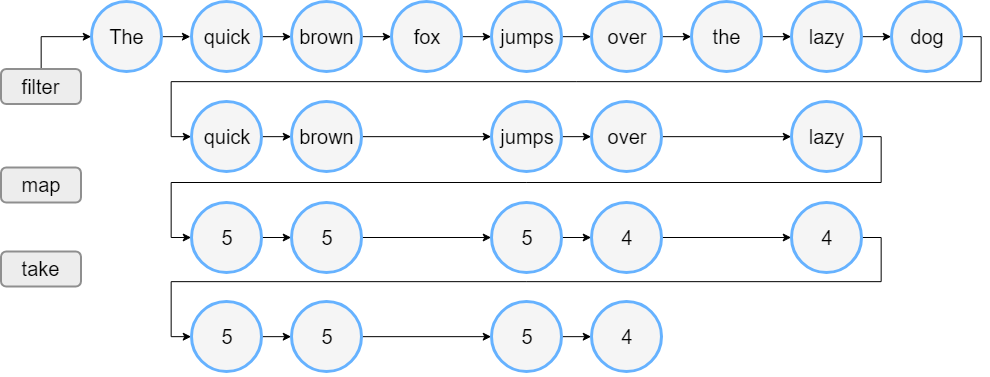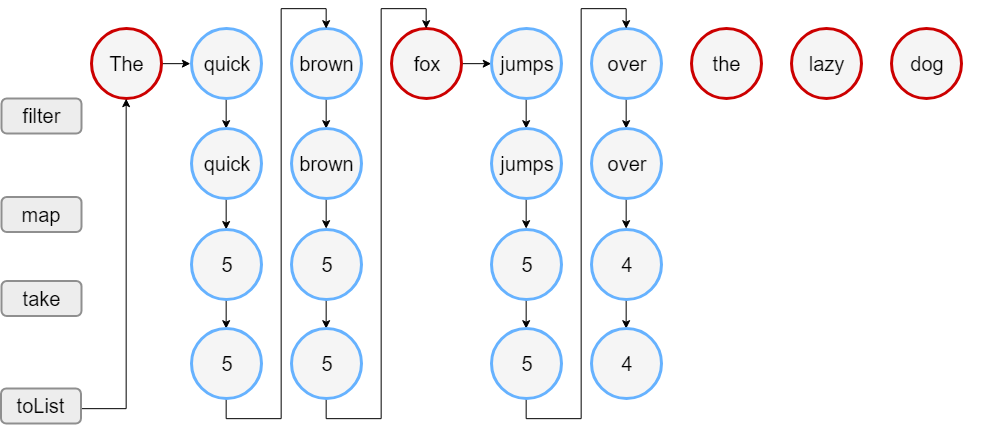Kotlin集合的创建与遍历

## 创建集合

``````val numbersSet = setOf("one", "two", "three", "four")
val emptySet = mutableSetOf<String>()``````

``val numbersMap = mapOf("key1" to 1, "key2" to 2, "key3" to 3, "key4" to 1)``

``val numbersMap = mutableMapOf<String, String>().apply { this["one"] = "1"; this["two"] = "2" }``

#### 空集合

``val empty = emptyList<String>()``

#### list 的初始化函数

``````val doubled = List(3, { it * 2 })  // 如果你想操作这个集合，应使用 MutableList
println(doubled)``````

#### 具体类型构造函数

``````val linkedList = LinkedList<String>(listOf("one", "two", "three"))
val presizedSet = HashSet<Int>(32)``````

#### 复制

``````val sourceList = mutableListOf(1, 2, 3)
val copyList = sourceList.toMutableList()
println("Copy size: \${copyList.size}")

``````val sourceList = mutableListOf(1, 2, 3)
val copySet = sourceList.toMutableSet()
println(copySet)``````

``````val sourceList = mutableListOf(1, 2, 3)
val referenceList = sourceList
println("Source size: \${sourceList.size}")``````

``````val sourceList = mutableListOf(1, 2, 3)
val referenceList: List<Int> = sourceList
println(referenceList) // 显示 sourceList 当前状态``````

#### 调用其他集合的函数

``````val numbers = listOf("one", "two", "three", "four")
val longerThan3 = numbers.filter { it.length > 3 }
println(longerThan3)``````

``````val numbers = setOf(1, 2, 3)
println(numbers.map { it * 3 })
println(numbers.mapIndexed { idx, value -> value * idx })``````

``````val numbers = listOf("one", "two", "three", "four")
println(numbers.associateWith { it.length })``````

## 迭代器

`Iterable<T>` 接口的继承者（包括 `Set``List`）可以通过调用 `iterator()` 函数获得迭代器。 一旦获得迭代器它就指向集合的第一个元素；

``````val numbers = listOf("one", "two", "three", "four")
val numbersIterator = numbers.iterator()
while (numbersIterator.hasNext()) {
println(numbersIterator.next())
}``````

``````val numbers = listOf("one", "two", "three", "four")
for (item in numbers) {
println(item)
}``````

``````val numbers = listOf("one", "two", "three", "four")
numbers.forEach {
println(it)
}``````

## List 迭代器

``````val numbers = listOf("one", "two", "three", "four")
val listIterator = numbers.listIterator()
while (listIterator.hasNext()) listIterator.next()
println("Iterating backwards:")
while (listIterator.hasPrevious()) {
print("Index: \${listIterator.previousIndex()}")
println(", value: \${listIterator.previous()}")
}``````

## 可变迭代器

``````val numbers = mutableListOf("one", "two", "three", "four")
val mutableIterator = numbers.iterator()

mutableIterator.next()
mutableIterator.remove()
println("After removal: \$numbers")``````

``````val numbers = mutableListOf("one", "four", "four")
val mutableListIterator = numbers.listIterator()

mutableListIterator.next()
mutableListIterator.next()
mutableListIterator.set("three")
println(numbers)``````

## 区间与数列

Kotlin 可通过调用 `kotlin.ranges` 包中的 `rangeTo()` 函数及其操作符形式的 `..` 轻松地创建两个值的区间。 通常，`rangeTo()` 会辅以 `in``!in` 函数。

``````if (i in 1..4) {  // 等同于 1 <= i && i <= 4
print(i)
}``````

``for (i in 1..4) print(i)``

``for (i in 4 downTo 1) print(i)``

``````for (i in 1..8 step 2) print(i)
println()
for (i in 8 downTo 1 step 2) print(i)``````

``````for (i in 1 until 10) {       // i in [1, 10), 10被排除
print(i)
}``````

## 区间

``````val versionRange = Version(1, 11)..Version(1, 30)
println(Version(0, 9) in versionRange)
println(Version(1, 20) in versionRange)``````

## 数列

``````for (int i = first; i <= last; i += step) {
// ……
}``````

``````for (i in 1..10)
print(i)``````

``````for (i in 1..8 step 2)
print(i)``````

• 对于正步长：不大于结束值且满足 `(last - first) % step == 0` 的最大值。
• 对于负步长：不小于结束值且满足 `(last - first) % step == 0` 的最小值。

``````for (i in 1..9 step 3)
print(i) // 最后一个元素是 7``````

``````for (i in 4 downTo 1)
print(i)``````

``println((1..10).filter { it % 2 == 0 })``

## 序列

`Iterable` 的处理包含多个步骤时，它们会优先执行：每个处理步骤完成并返回其结果——中间集合。 在此集合上执行以下步骤。

## 构造一个序列，主要有以下方法

#### 由元素

``val numbersSequence = sequenceOf("four", "three", "two", "one")``

#### 由 Iterable

``````val numbers = listOf("one", "two", "three", "four")

#### 由函数

``````val oddNumbers = generateSequence(1) { it + 2 } // `it` 是上一个元素
println(oddNumbers.take(5).toList())
//println(oddNumbers.count())     // 错误：此序列是无限的。``````

``````val oddNumbersLessThan10 = generateSequence(1) { if (it + 2 < 10) it + 2 else null }
println(oddNumbersLessThan10.count())``````

#### 由组块

`yieldAll()``Sequence` 参数可以是无限的。 当然，这样的调用必须是最后一个：之后的所有调用都永远不会执行。

``````val oddNumbers = sequence {
yield(1)
yieldAll(listOf(3, 5))
yieldAll(generateSequence(7) { it + 2 })
}
println(oddNumbers.take(5).toList())``````

#### 序列操作

• 无状态 操作不需要状态，并且可以独立处理每个元素，例如 map() 或 filter()。 无状态操作还可能需要少量常数个状态来处理元素，例如 take() 与 drop()。
• 有状态 操作需要大量状态，通常与序列中元素的数量成比例。

#### 序列处理示例

Iterable 假定有一个单词列表。下面的代码过滤长于三个字符的单词，并打印前四个单词的长度。

``````val words = "The quick brown fox jumps over the lazy dog".split(" ")
val lengthsList = words.filter { println("filter: \$it"); it.length > 3 }
.map { println("length: \${it.length}"); it.length }
.take(4)

println("Lengths of first 4 words longer than 3 chars:")
println(lengthsList)``````Sequence 现在用序列写相同的逻辑：

``````val words = "The quick brown fox jumps over the lazy dog".split(" ")
// 将列表转换为序列
val wordsSequence = words.asSequence()

val lengthsSequence = wordsSequence.filter { println("filter: \$it"); it.length > 3 }
.map { println("length: \${it.length}"); it.length }
.take(4)

println("Lengths of first 4 words longer than 3 chars")
// 末端操作：以列表形式获取结果。
println(lengthsSequence.toList())``````Lv1

88

34

83